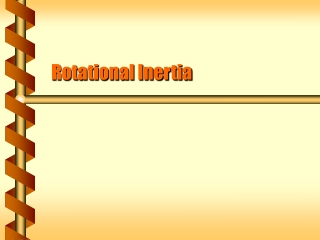DownloadDownload PresentationRotational Inertia

# Rotational Inertia

Télécharger la présentation## Rotational Inertia

- - - - - - - - - - - - - - - - - - - - - - - - - - - E N D - - - - - - - - - - - - - - - - - - - - - - - - - - -
##### Presentation Transcript

1. Rotational Inertia

2. Real objects have mass at points other than the center of mass. Each point in an object can be measured from an origin at the center of mass. If the positions are fixed compared to the center of mass it is a rigid body. Rigid Body ri

3. The motion of a rigid body includes the motion of its center of mass. This is translational motion A rigid body can also move while its center of mass is fixed. This is rotational motion. Translation and Rotation vCM 

4. Circular Motion • Objects in circular motion have kinetic energy. • K = ½ m v2 • The velocity can be converted to angular quantities. • K = ½ m (rw)2 • K = ½ (mr2) w2 m r w

5. Integrated Mass • The kinetic energy is due to the kinetic energy of the individual pieces. • The form is similar to linear kinetic energy. • KCM = ½ mv2 • Krot = ½ Iw2 • The term Iis the moment of inertia of a particle.

6. Moment of Inertia Defined • The moment of inertia measures the resistance to a change in rotation. • Mass measures resistance to change in velocity • Moment of inertia I = mr2 for a single mass • The total moment of inertia is due to the sum of masses at a distance from the axis of rotation.

7. A spun baton has a moment of inertia due to each separate mass. I = mr2 + mr2 = 2mr2 If it spins around one end, only the far mass counts. I = m(2r)2 = 4mr2 Two Spheres m m r

8. Extended objects can be treated as a sum of small masses. A straight rod (M) is a set of identical masses Dm. The total moment of inertia is Each mass element contributes The sum becomes an integral Mass at a Radius distance r to r+Dr length L axis

9. Rigid Body Rotation • The moments of inertia for many shapes can found by integration. • Ring or hollow cylinder: I= MR2 • Solid cylinder: I= (1/2)MR2 • Hollow sphere: I= (2/3)MR2 • Solid sphere: I= (2/5)MR2

10. The point mass, ring and hollow cylinder all have the same moment of inertia. I= MR2 All the mass is equally far away from the axis. The rod and rectangular plate also have the same moment of inertia. I= (1/3) MR2 The distribution of mass from the axis is the same. Point and Ring M R R M M M length R length R axis

11. Some objects don’t rotate about the axis at the center of mass. The moment of inertia depends on the distance between axes. The moment of inertia for a rod about its center of mass: Parallel Axis Theorem h = R/2 M axis

12. How much energy is stored in the spinning earth? The earth spins about its axis. The moment of inertia for a sphere: I = 2/5 MR2 The kinetic energy for the earth: Krot = 1/5 MR2w2 With values: K = 2.56 x 1029 J Spinning Energy The energy is equivalent to about 10,000 times the solar energy received in one year.